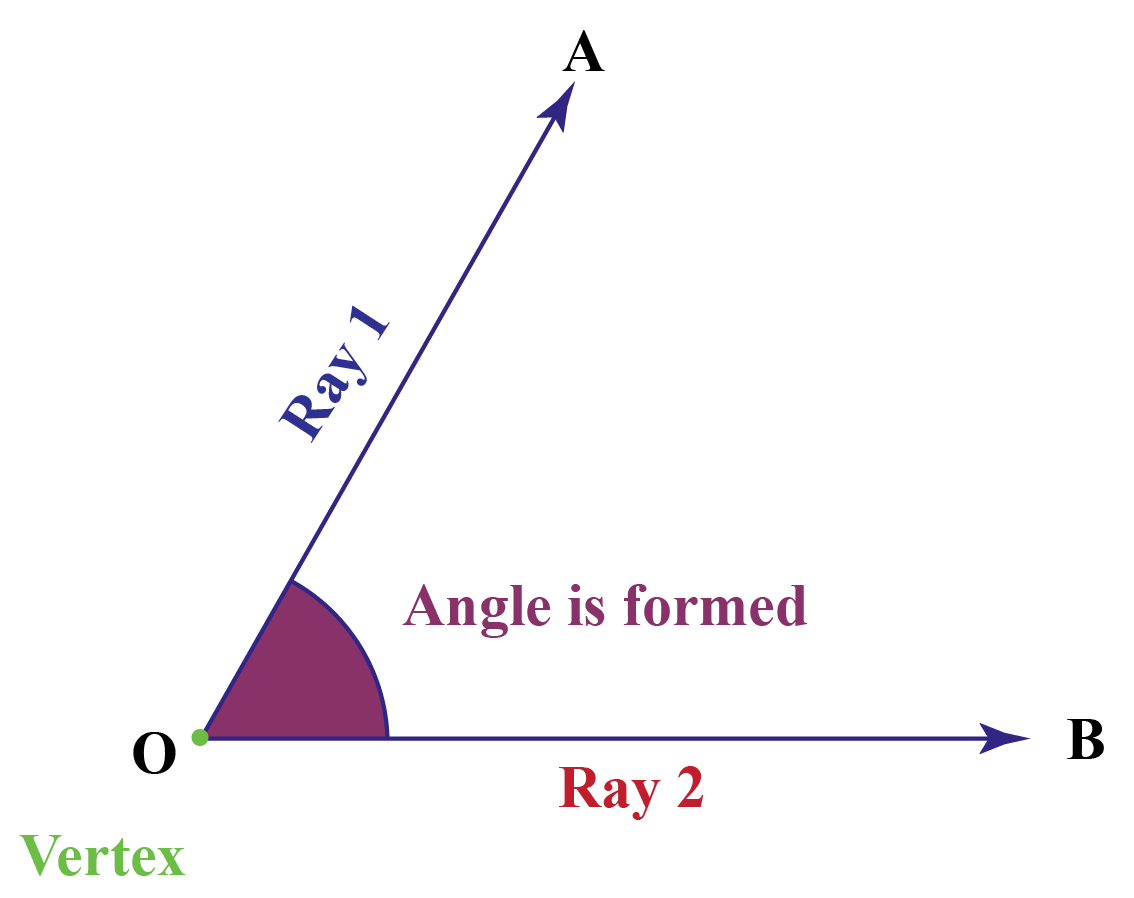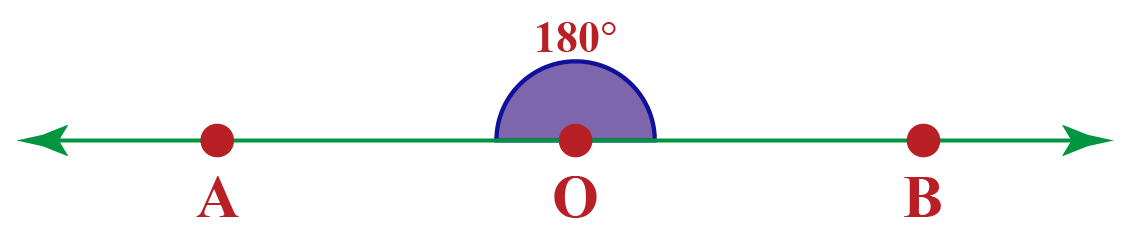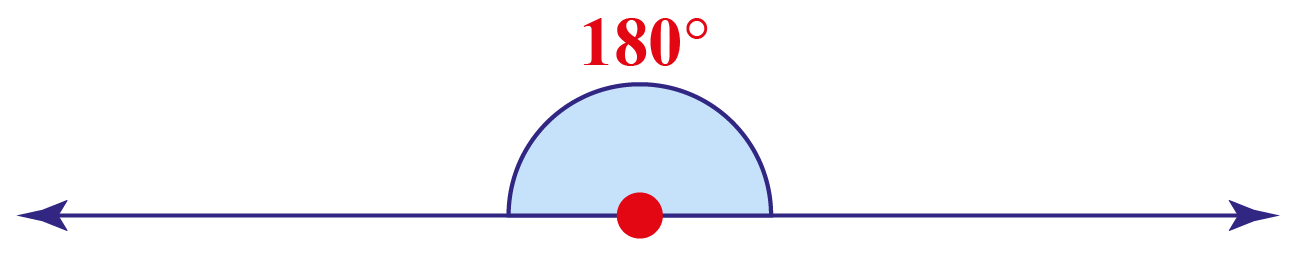# 180 degree angle

180 degree angle
Go back to  'Angles'

In this mini-lesson, we will learn about 180-degree angles. While doing so we will also discuss creating a 180-degree angle with a compass, and steps to be followed to construct a 180-degree angle. You can check out the interactive simulations to know more about the lesson and try your hand at solving a few interesting practice questions at the end of the page.

Before we get started, have you ever noticed the angle between the two hands of a clock when at 6 o'clock?The angle between the two hands of the clock above is 180° because it forms a straight line.

Let us explore more about the 180° angle.

## Lesson Plan

 1 What is 180-Degree Angle? 2 Thinking out of the Box! 3 Solved Examples on 180-Degree Angle 4 Important Notes on 180-Degree Angle 5 Interactive Questions on 180-Degree Angle

## What Is 180 Degree Angle?

We know that an angle is formed when two rays meet at a vertex.If the angle formed at the vertex O measures 180°, we call it a 180-degree angle.

A straight angle is called a 180-degree angle.

It is also called a half-circle. It looks like a straight line.## How to Construct 180-Degree Angle Using a Protractor?

In this section, let us explore how to construct a 180-degree angle with the help of a protractor.

• Step 1: Draw a line segment $$OA$$
• Step 2: Place the protractor at the point $$O$$
• Step 3: In the outer circle of the protractor look for 180° reading and with a pencil mark a dot and name it $$C$$
• Step 4: Join $$O$$ and $$C$$. Now,  $$\angle AOC =180 ^\circ$$

Still, confused? Look at the simulation below.

Click on the "Go" button to understand the process of construction of a 180-degree angle using a protractor.

## What Are 180-degree Angles in Real Life?

180° angles are widely seen in real life.

In everyday life, 180-degree angles are used. For constructing structures, highways, houses, and sports facilities, engineers and architects use angles. Carpenters make benches, tables, and sofas using angles.Observe where you can see 180° angles in your surrounding areas.Think Tank
• How many 180-degree angles are there in a complete angle?

## Solved Examples

 Example 1

Can you help Alex find out the difference between a 180° angle and a 90° angle?

Solution

The 180-degree angle is a straight line, known as a semi-circle.

A 90-degree angle is a quadrant or one-fourth of a circle.

 180-degree is a straight line or semicircle and 90 degree is a quadrant
 Example 2

Alina wants to construct a 180° angle using a protractor. Can you help her construct?

Solution

Step 1: Draw a ray (part of a line that has a vertex at one end and an arrowhead at another). Mark the vertex as point O.

Step 2: Point O denotes the vertex of the angle.

Step 3: Now put the protractor above the point O vertex and match the baseline of the protractor along with the arm of the angle.

Step 4: Mark the angle 180 degrees with a dot.

Step 5: Now join this new vertex with point O. This forms another arm of the angle.

Now, we get a straight angle.More Important Topics
Numbers
Algebra
Geometry
Measurement
Money
Data
Trigonometry
Calculus